# 大前端

## 1 简介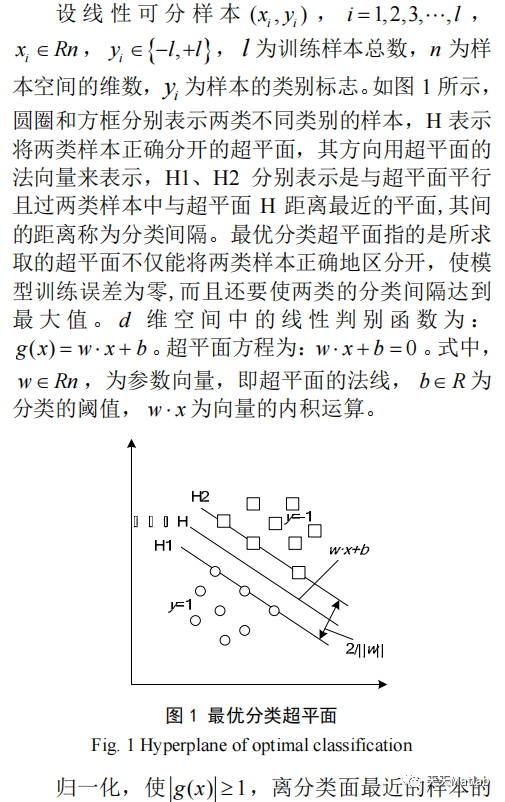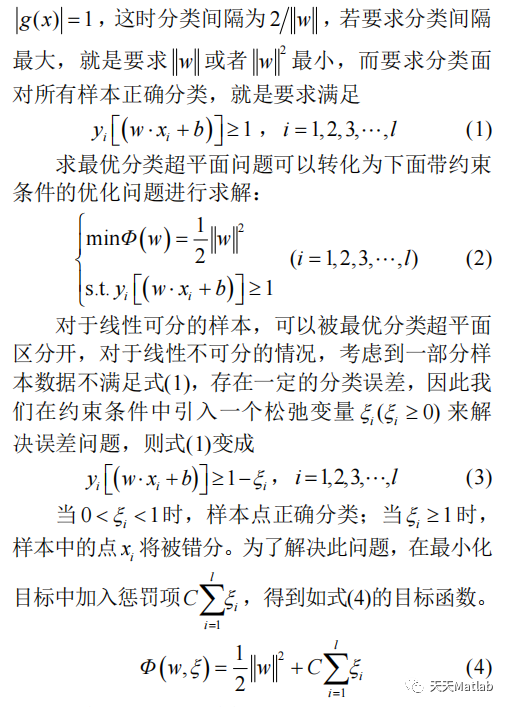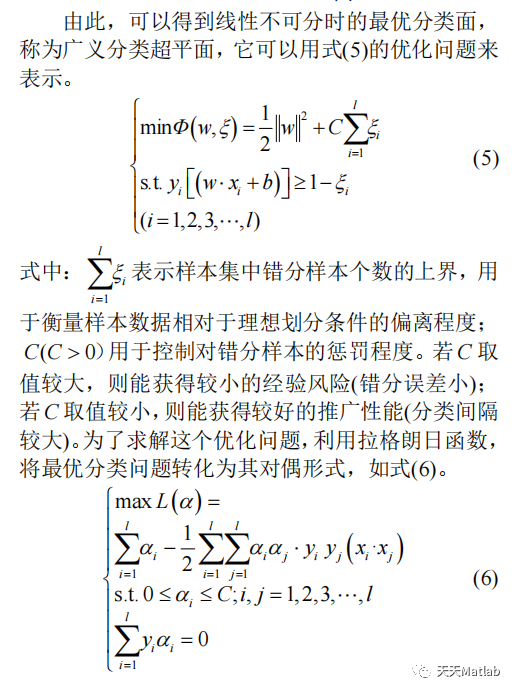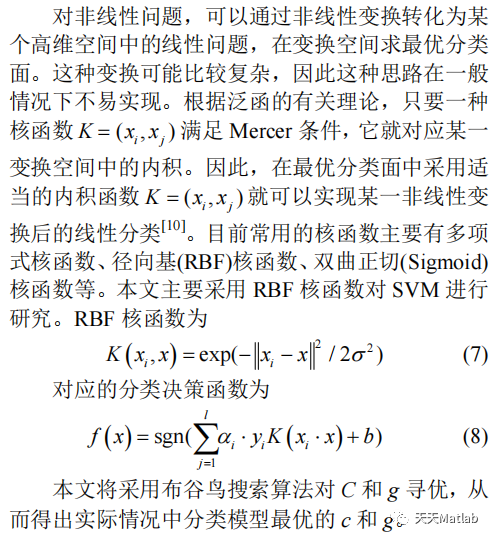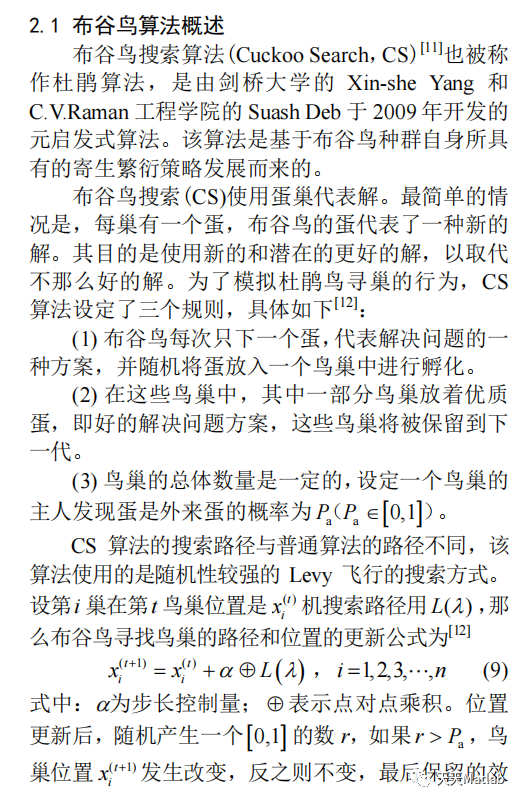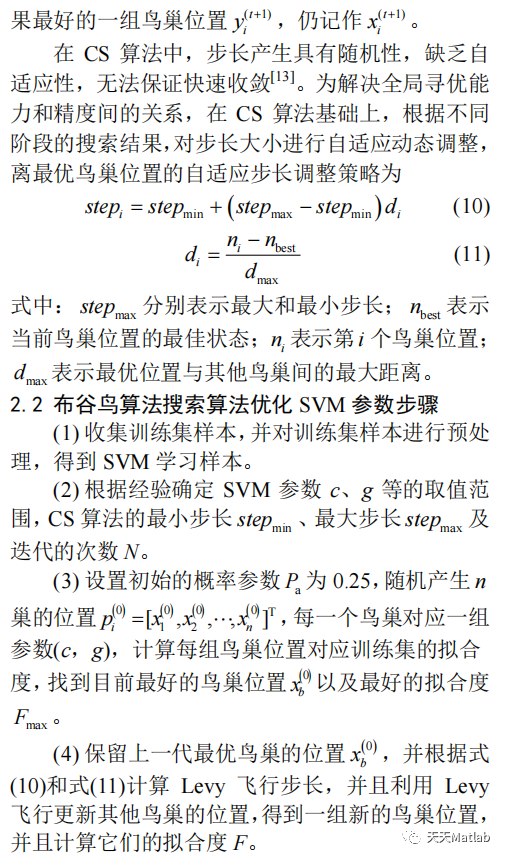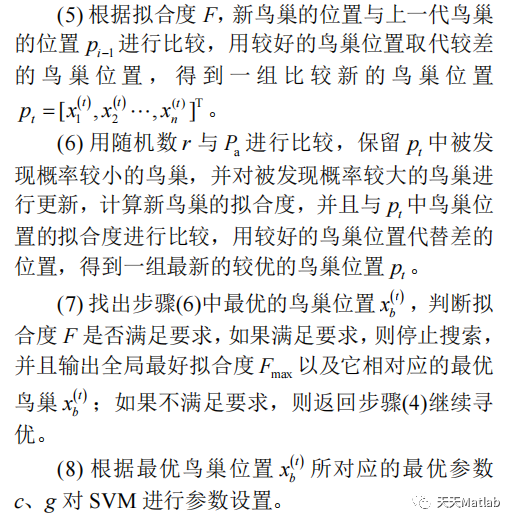## 2 部分代码

```%% 数据的提取和预处理

% 载入测试数据上证指数(1990.12.19-2009.08.19)
% 数据是一个4579*6的double型的矩阵,每一行表示每一天的上证指数
% 6列分别表示当天上证指数的开盘指数,指数最高值,指数最低值,收盘指数,当日交易量,当日交易额.
clear
clc

% 提取数据
[m,n] = size(sh);
ts = sh(2:m,1);    % 选取2到4579个交易日内每日的开盘指数作为因变量
tsx =sh(1:m-1,:); %选取1到4578个交易日

% 数据预处理,将原始数据进行归一化
ts = ts';
tsx = tsx';

% mapminmax为matlab自带的映射函数
% 对ts进行归一化
[TS,TSps] = mapminmax(ts,1,2);%归一化在区间[1 2]
% 对TSX进行转置,以符合libsvm工具箱的数据格式要求
TS = TS';

% mapminmax为matlab自带的映射函数
% 对tsx进行归一化
[TSX,TSXps] = mapminmax(tsx,1,2);%归一化在区间[1 2]
% 对TSX进行转置,以符合libsvm工具箱的数据格式要求
TSX = TSX';

Tol=1.0e-5;
n=25;%鸟巢个数
% Discovery rate of alien eggs/solutions
pa=0.25;

%为最大迭代次数限制
%% Simple bounds of the search domain
% Lower bounds
nd=2;
Lb=0.01*ones(1,nd);
% Upper bounds
Ub=100*ones(1,nd);                                                              %随机产生初始解
% Random initial solutions
for i=1:n,
nest(i,:)=Lb+(Ub-Lb).*rand(size(Lb));
end
%得到当前的最优解
% Get the current best
for i=1:n
fitness(i)=fun(nest(i,:));
end

N_iter=0;                                                                   %开始迭代
%% Starting iterations
for iter=1:1 %while (fmin>Tol),

bestc=bestnest(1);
bestg=bestnest(2);

cmd = ['-c ', num2str(bestc), ' -g ', num2str(bestg) , ' -s 3 -p 0.01'];
model = svmtrain(TS,TSX,cmd);

%% SVM网络回归预测
[predict,mse,~] = svmpredict(TS,TSX,model);%课本138页没有prob_estimates
predict = mapminmax('reverse',predict',TSps); %反归一化
predict = predict';
error=predict-ts';
errorn=sum(abs(error));
figure;
hold on;
plot(ts,'-o');
plot(predict,'r-^');
legend('原始数据','回归预测数据');
hold off;
title('原始数据和回归预测数据对比','FontSize',12);
xlabel('交易日天数(1990.12.19-2009.08.19)','FontSize',12);
ylabel('开盘数','FontSize',12);
grid on;
img =gcf;  %获取当前画图的句柄
print(img, '-dpng', '-r600', './img.png')         %即可得到对应格式和期望dpi的图像```

## 3 仿真结果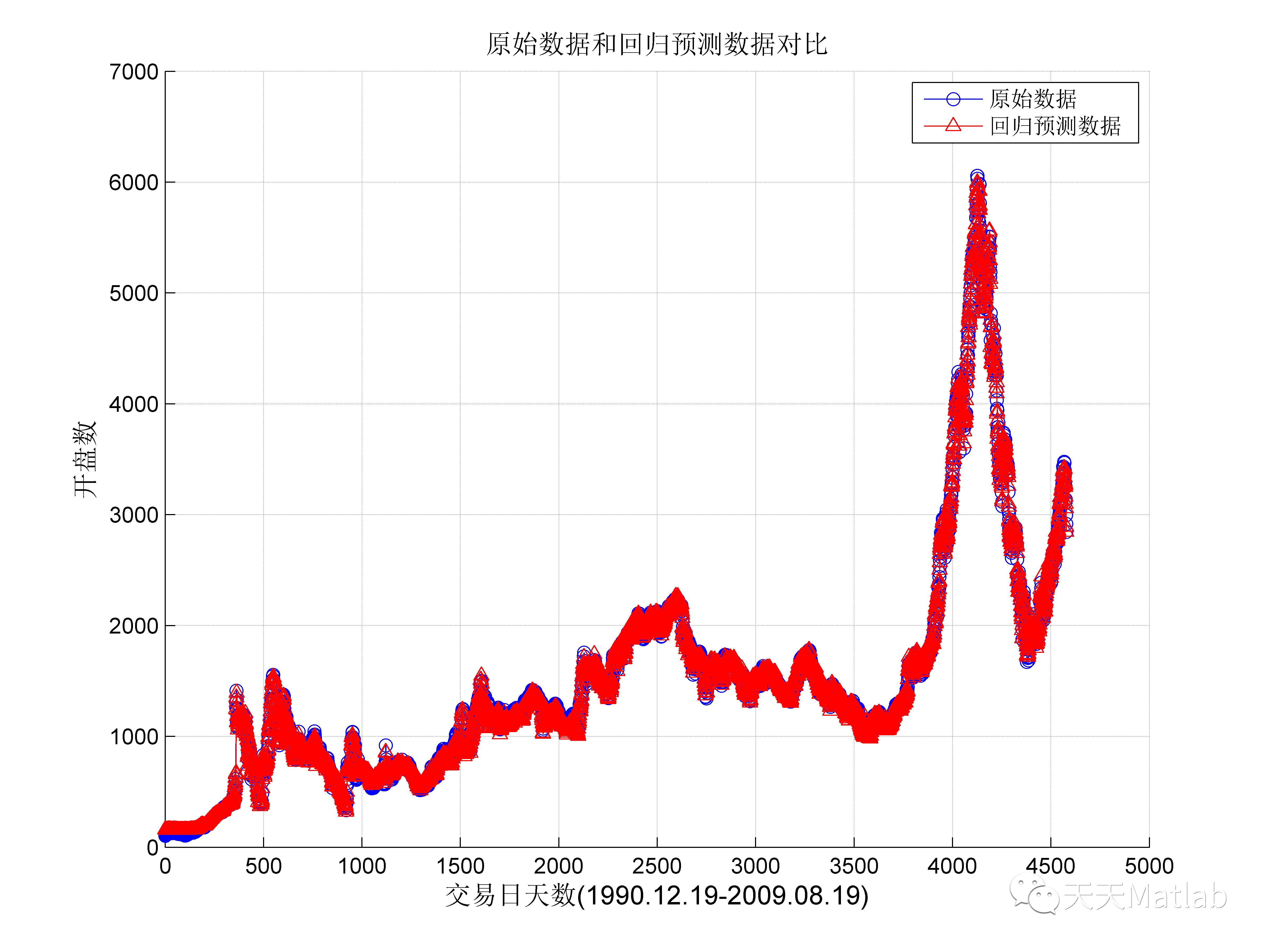## 4 参考文献

路璐, and 程良伦. "改进布谷鸟搜索算法优化SVM的网络流量预测模型." 计算机应用与软件 01(2015):124-127.友情链接